Created June 24, 2016 © GPL3+

# IntelliBin (The Smart Trash Bin)

Despite initiatives to teach people good recycling habits, recyclables are still being improperly disposed. IntelliBin aims to fix that.

IntermediateFull instructions provided24 hours891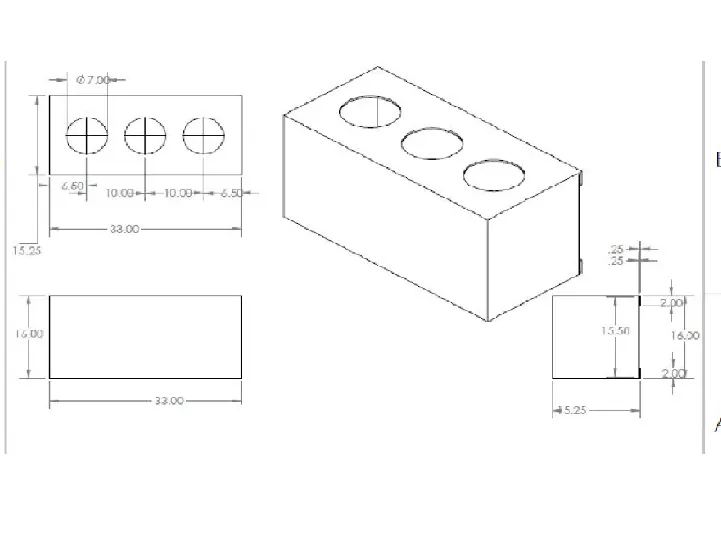## Things used in this project

### Hardware componentsRaspberry Pi 3 Model B
×1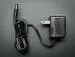9V 1A Switching Wall Power Supply
×1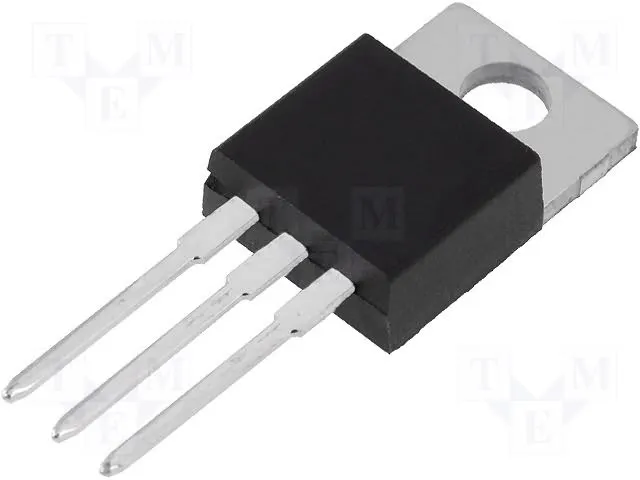Linear Regulator (7805)
×1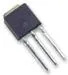Power MOSFET N-Channel
×1
 logitech c270 webcam
×1

### Software apps and online services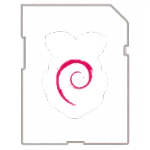Raspberry Pi Raspbian

### Hand tools and fabrication machinesSoldering iron (generic)
 Table Saw

## Custom parts and enclosures

### Enclosure dimensions.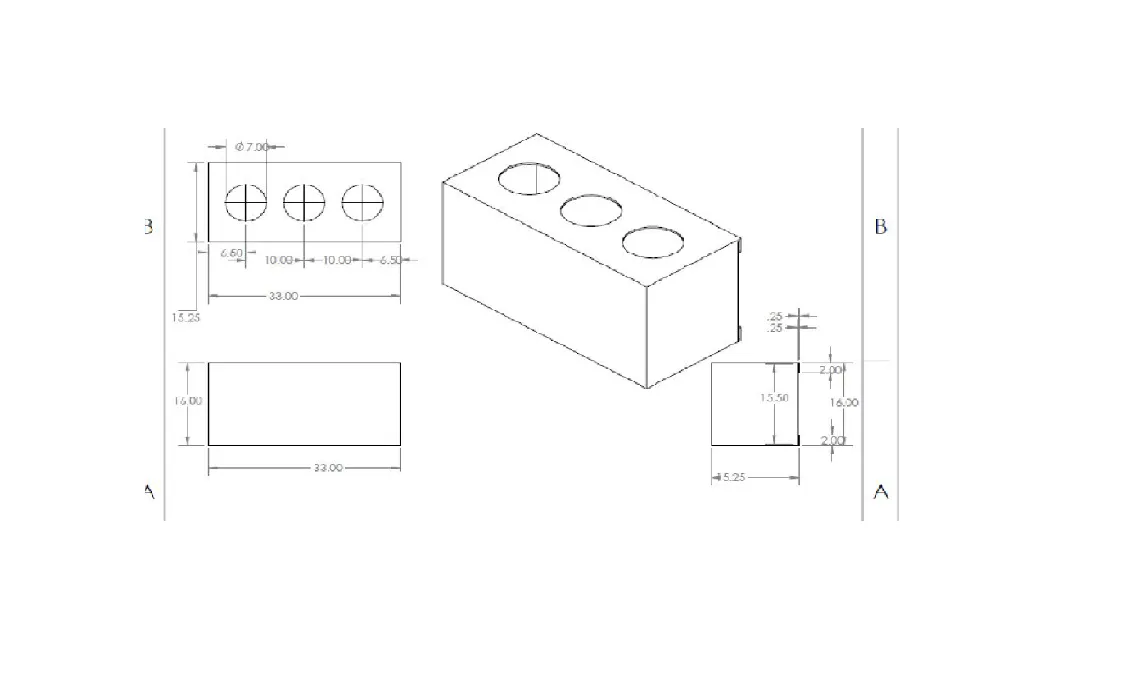## Schematics

### Simple Overall Diagram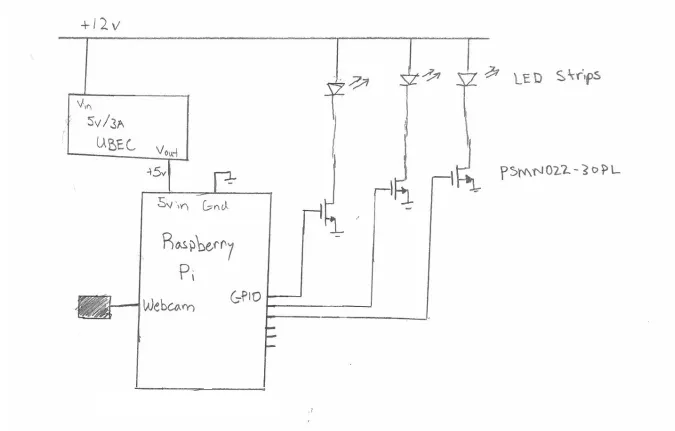### Flow Chart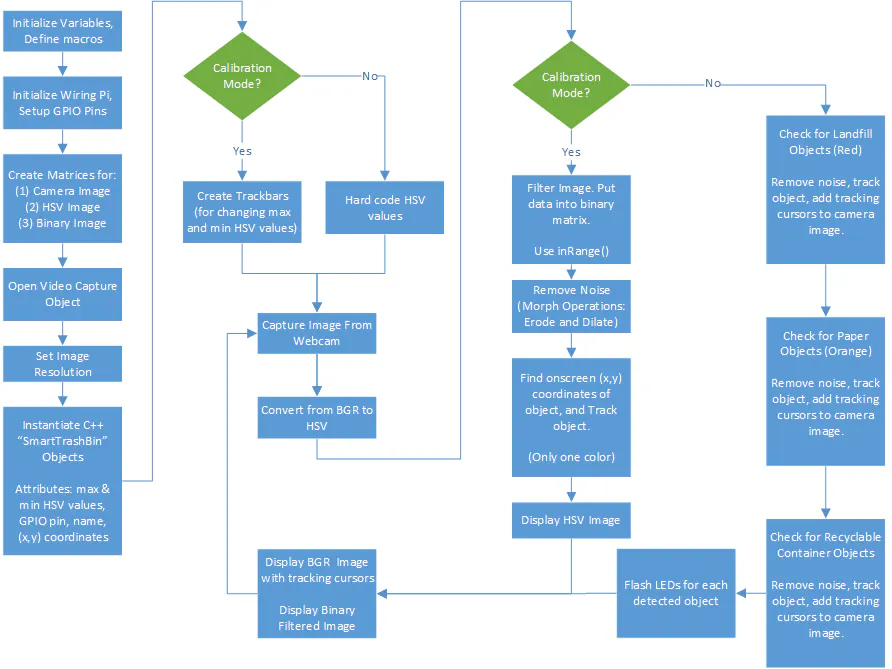### Timeline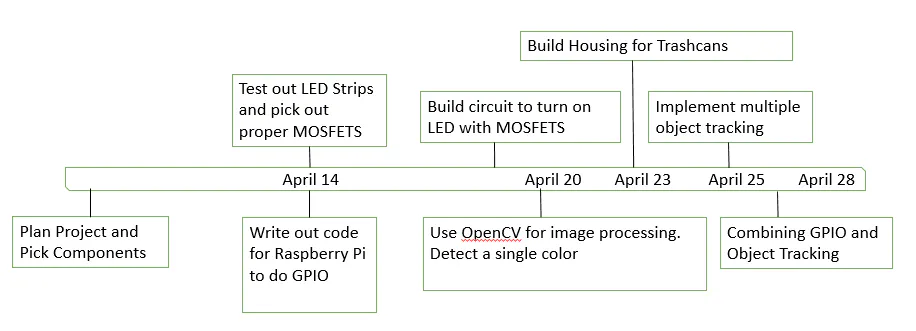## Code

### Main.c

C/C++
Run it from a Raspberry Pi

Compile:
g++ -ggdb `pkg-config --cflags opencv` -o `basename main.cpp .cpp` main.cpp SmartTrashBinObjects.cpp `pkg-config --libs opencv`

Run:
sudo ./main
```//#include <opencv2/highgui/highgui.hpp> //Including in SmartTrashBinObjects.h for the sake of organization
//#include <opencv/cv.hpp> //included in SmartTrashBinObjects.h to avoid redundancy and improve compile time

#include <string>
#include <sstream>
#include <iostream>
#include <stdio.h>

#include <wiringPi.h>

#include "SmartTrashBinObjects.h"

#include <vector>

//using namespace cv;
//using namespace std;

//build using: g++ -ggdb `pkg-config --cflags opencv` -o `basename main.cpp .cpp` main.cpp `pkg-config --libs opencv`

//UPDATE: build using this to incorporate the SmartTrashBinObjects.cpp file:
//g++ -ggdb `pkg-config --cflags opencv` -o `basename main.cpp .cpp` main.cpp SmartTrashBinObjects.cpp `pkg-config --libs opencv`

//STEP 1 convert from BGR(blue, green, red) to HSV color space (hue, saturation, value )

#define FRAME_WIDTH		400//640
#define FRAME_HEIGHT 		300//480

#define MAX_NUM_OBJECTS 	7

#define MIN_OBJECT_AREA	45*45
#define MAX_OBJECT_AREA	FRAME_HEIGHT*FRAME_WIDTH/1.5

//going to use these values in a trackbar (max and min HSV colorspace values)
int H_MIN = 0, H_MAX = 256;
int S_MIN = 0, S_MAX = 256;
int V_MIN = 0, V_MAX = 256;

//Window names
const string
windowName 	= "Original Image",
windowName1 	= "HSV Image",
windowName2 	= "Threshold Image",
windowName3	= "After Morphological Operations",
trackbarWindowName = "Trackbars";

string IntToString(int number)
{
std::stringstream ss;
ss << number;
return ss.str();
}

void on_trackbar(int, void*)
{
//Nothing
}

void CreateTrackbars()
{
//create a window
namedWindow(trackbarWindowName, 0);

//create memory to store tackbar name on window
char TrackbarName;

sprintf(TrackbarName, "H_MIN", H_MIN);
sprintf(TrackbarName, "H_MAX", H_MAX);

sprintf(TrackbarName, "S_MIN", S_MIN);
sprintf(TrackbarName, "S_MAX", S_MAX);

sprintf(TrackbarName, "V_MIN", V_MIN);
sprintf(TrackbarName, "V_MAX", V_MAX);

//create and insert trackbars into window
//createTrackbar(Name to appear beside trackbar, name of window to appear in, address of modifyable value, max value, function to call whenever something is changed.)

createTrackbar( "H_MIN", trackbarWindowName, &H_MIN, H_MAX, on_trackbar);
createTrackbar( "H_MAX", trackbarWindowName, &H_MAX, H_MAX, on_trackbar);

createTrackbar( "S_MIN", trackbarWindowName, &S_MIN, S_MAX, on_trackbar);
createTrackbar( "S_MAX", trackbarWindowName, &S_MAX, S_MAX, on_trackbar);

createTrackbar( "V_MIN", trackbarWindowName, &V_MIN, V_MAX, on_trackbar);
createTrackbar( "V_MAX", trackbarWindowName, &V_MAX, V_MAX, on_trackbar);

}

//void DrawObject(int x, int y, Mat &frame) //instead of passing in ints x and y, we'll pass in an c++ object we created
//void DrawObject(Waste ourWaste, Mat &frame) //instead of passing in a single object,  we're gonna pass in a vector containing Waste objects (corrected line is below)
void DrawObject(vector<Waste> ourWaste, Mat &frame)
{
//unpacking the 'ourWaste' vector, containing all the similar objects we see on screen
for(int i = 0; i < ourWaste.size(); i++)
{
//immediately below is code for a single object detection algorithm
//circle(frame, Point(x,y), 20, Scalar(0,255,0), 2);
//int x = ourWaste.getXPos();
//int y = ourWaste.getYPos();

int x = ourWaste.at(i).getXPos();
int y = ourWaste.at(i).getYPos();

circle(frame, Point(x,y), 20, Scalar(0,255,0), 2);
//putText(frame to put text on, TEXT, font face, size i think, color)
putText(frame, IntToString(x) + " , " + IntToString(y), Point(x, y+20), 1, 1, Scalar(0, 255, 0)); //an offset of +20 for our y value means we're going downward on the screen

//new! This is the text we will put next to the object to identify the type of trash we will be putting into it
putText(frame,  ourWaste.at(i).getType(), Point(x, y-30), 1, 2, ourWaste.at(i).getTextColor() );
}
}

void MorphOps(Mat &thresh)
{
//we want to erode and dilate the image

//these are the structuring elements that will be used to do this
//the erode element will be a 3x3 rectangle
Mat erodeElement = getStructuringElement(MORPH_RECT, Size(3, 3));
//we will dilate with a larger element to make sue object is nicely visible
Mat dilateElement = getStructuringElement(MORPH_RECT, Size(8, 8));

erode(thresh,thresh,erodeElement);
erode(thresh,thresh,erodeElement);
erode(thresh,thresh,erodeElement);

dilate(thresh, thresh, dilateElement);
dilate(thresh, thresh, dilateElement);
}

//We can actually overload this function and implement a different version of it so that it can take in a different combination of parameters
//I've implemented the 2nd version of this method below this immediate one
void TrackFilteredObject(int &x, int&y, Mat threshold, Mat &cameraFeed)
{

//int x, y; //instead of using just pure x and y positions, we're gonna use classes

//Waste paper; //object named paper of class Waste,  from SmartTrashBinObjects.h

//creating a vector to contain the multiple paper objects we're gonna be seeing on the camera
//this is essentially an array that allocates more space than we need so that we can push stuff onto it easily
vector<Waste> paperObjects; //this should just be a generic waste object, Idk why i named it paper objects

Mat temp;
threshold.copyTo(temp);

vector <vector <Point> > contours;
vector <Vec4i> hierarchy;

findContours(temp, contours, hierarchy, CV_RETR_CCOMP, CV_CHAIN_APPROX_SIMPLE);

double refArea = 0;
bool objectFound = false;

if(hierarchy.size() > 0)
{
int numObjects = hierarchy.size();
if(numObjects < MAX_NUM_OBJECTS)
{
for (int index = 0; index >= 0; index = hierarchy[index])
{
Moments moment = moments((cv::Mat)contours[index]);
double area = moment.m00;

if(area > MIN_OBJECT_AREA) //&& area <MAX_OBJECT_AREA && area > refArea - ADDITIONAL UNECESSARY CONDITIONS
{
//Waste.xPos = moment.m10/area; //won't work, xPos is private. Need to create a function to write values to it.

//private variables are used in large programs to localize variables that many objects have (like xPos or yPos in our case) to those SPECIFIC objects

//x = moment.m10/area;
//y = moment.m01/area;

//need to create a temporary paper object of class Waste
Waste paper;

//Set x and y position of  paper object
//paper.setXPos(moment.m10/area);
//paper.setYPos(moment.m01/area);

paper.setXPos(moment.m10/area);
paper.setYPos(moment.m01/area);

//push this new object into our paperObjects vector. This allows us to have multiple instances of it
paperObjects.push_back(paper);

objectFound = true;
refArea = area;
}
else
{
objectFound = false;
}
}

if(objectFound)
{
putText(cameraFeed, "Tracking Object", Point(0,50),2,1, Scalar(0,255,0), 2);
//DrawObject(x,y,cameraFeed); //trying to eliminate usage of x and y

//DrawObject(paper,cameraFeed); //instead of drawing just one object like in this line, we want to draw the entire vector.
DrawObject(paperObjects,cameraFeed); //this will draw all of the objects we pushed onto the paperObjects vector

}
else
{
putText(cameraFeed, "No objects found. Adjust Filter.", Point(0,50), 1,2,Scalar(0,0,255, 2));
}
}
}
}

//2nd version of TrackFilteredObject. Now designed to receive an object
void TrackFilteredObject(Waste ourWaste, Mat threshold, Mat HSV, Mat &cameraFeed)
{

//int x, y; //instead of using just pure x and y positions, we're gonna use classes

//Waste paper; //object named paper of class Waste,  from SmartTrashBinObjects.h

//creating a vector to contain the multiple paper objects we're gonna be seeing on the camera
//this is essentially an array that allocates more space than we need so that we can push stuff onto it easily
vector<Waste> paperObjects;

Mat temp;
threshold.copyTo(temp);

vector <vector <Point> > contours;
vector <Vec4i> hierarchy;

findContours(temp, contours, hierarchy, CV_RETR_CCOMP, CV_CHAIN_APPROX_SIMPLE);

double refArea = 0;
bool objectFound = false;

if(hierarchy.size() > 0)
{
int numObjects = hierarchy.size();
if(numObjects < MAX_NUM_OBJECTS)
{
for (int index = 0; index >= 0; index = hierarchy[index])
{
Moments moment = moments((cv::Mat)contours[index]);
double area = moment.m00;

if(area > MIN_OBJECT_AREA)
{
//Waste.xPos = moment.m10/area; //won't work, xPos is private. Need to create a function to write values to it.

//private variables are used in large programs to localize variables that many objects have (like xPos or yPos in our case) to those SPECIFIC objects

//x = moment.m10/area;
//y = moment.m01/area;

//need to create a temporary paper object of class Waste
Waste paper;

//Set x and y position of  paper object
//paper.setXPos(moment.m10/area);
//paper.setYPos(moment.m01/area);

paper.setXPos(moment.m10/area);
paper.setYPos(moment.m01/area);

//new things implemented to set our Text and color of our text
paper.setType(ourWaste.getType());
paper.setTextColor(ourWaste.getTextColor());

//push this new object into our paperObjects vector. This allows us to have multiple instances of it
paperObjects.push_back(paper);

objectFound = true;
}
else
{
objectFound = false;
}
}

if(objectFound)
{
putText(cameraFeed, "Tracking Object", Point(0,50),2,1, Scalar(0,255,0), 2);
//DrawObject(x,y,cameraFeed); //trying to eliminate usage of x and y

//DrawObject(paper,cameraFeed); //instead of drawing just one object like in this line, we want to draw the entire vector.
DrawObject(paperObjects,cameraFeed); //this will draw all of the objects we pushed onto the paperObjects vector

}
else
{
putText(cameraFeed, "No objects found. Adjust Filter.", Point(0,50), 1,2,Scalar(0,0,255, 2));
}
}
}
}

int main(int argc, char* argv[])
{
bool calibrationMode 		= false;
bool trackObjects 		= true;
bool useMorphOperations 	= true;

//Create matrix to store each frame of the webcam feed
Mat cameraFeed;

//Create matrix to store HSV image
Mat HSV;

//Create matrix to store binary threshold image (black/white)
Mat threshold;

//Used for image tracking
int xObject = 0, yObject = 0;

//Creates the sliders/trackbars to allow us to select values for HSV filtering
if(calibrationMode)
{
CreateTrackbars();
}
/*
H_MIN = 0;
H_MAX = 256;
S_MIN = 174;
S_MAX = 256;
V_MIN = 113;
V_MAX = 256;
*/

//Create a VideoCapture object. This basically means get a video image from the camera
VideoCapture capture;

//open capture object at location zero (ie get media from the camera device 0)
capture.open(0);

//seting height and width of capture frame
capture.set(CV_CAP_PROP_FRAME_WIDTH, FRAME_WIDTH);
capture.set(CV_CAP_PROP_FRAME_HEIGHT, FRAME_HEIGHT);

//where all the work is done
while(1)
{
//read data from camera and store into CameraFeed matrix

//converts from one color space to another (in our case BGR to HSV colorspace)
//cvtColor(input image frame, output frame, colorspace conversion code, number of channels in output img)
cvtColor(cameraFeed, HSV, COLOR_BGR2HSV); // cameraFeed ---BGR to HSV--> HSV

if(calibrationMode)
{

//filter HSV image and display output to the threshold matrix
//inRange(input array, lowerBound array, upperBound array, output array)
//sees is the values in the HSV matrix lie within the desired bounds, and outputs a 1 in the respective index in
//the output array, threshold, which is how we will see the object
inRange(HSV, Scalar(H_MIN,S_MIN,V_MIN), Scalar(H_MAX,S_MAX,V_MAX), threshold);

//eliminate noise through morphological operations on threshold image
if(useMorphOperations)
{
MorphOps(threshold);
}

imshow(windowName2, 	threshold);

if(trackObjects)
{
TrackFilteredObject(xObject, yObject, threshold, cameraFeed);
}
}
else //if not in calibration mode, hardcode our values in!!!
{
Waste orange("orange"), papers("paper"), indomie("plastic");

//hardcoding values for each of our objects here
//calibrated for orange paper
orange.setHSVmin(Scalar(0, 111, 159));
orange.setHSVmax(Scalar(58, 194, 256));

//calibrated for purple  paper
papers.setHSVmin(Scalar(120, 68, 144));
papers.setHSVmax(Scalar(153, 120, 187));

//calibrated for red paper
indomie.setHSVmin(Scalar(160, 79, 192));
indomie.setHSVmax(Scalar(175, 256, 256));

//filter HSV image and display output to the threshold matrix
//inRange(input array, lowerBound array, upperBound array, output array)
//sees is the values in the HSV matrix lie within the desired bounds, and outputs a 1 in the respective index in
//the output array, threshold, which is how we will see the object
inRange(HSV, orange.getHSVmin(), orange.getHSVmax(), threshold);
MorphOps(threshold);
TrackFilteredObject(orange, threshold, HSV, cameraFeed);
//TrackFilteredObject(xObject, yObject, threshold, cameraFeed);

inRange(HSV, papers.getHSVmin(), papers.getHSVmax(), threshold);
MorphOps(threshold);
//TrackFilteredObject(xObject, yObject, threshold, cameraFeed);
TrackFilteredObject(papers, threshold, HSV, cameraFeed);

inRange(HSV, indomie.getHSVmin(), indomie.getHSVmax(), threshold);
MorphOps(threshold);
//TrackFilteredObject(xObject, yObject, threshold, cameraFeed); //sort of deprecated use of the TrackFilteredObject method
TrackFilteredObject(indomie, threshold, HSV, cameraFeed);

}

//Show original image, HSV'd image, and the filtered image
//imshow(windowName2, 	threshold);
imshow(windowName, 	cameraFeed);
//imshow(windowName1,	HSV);

//we need this, images won't appear without this function here.
waitKey(30);
}

return 0;

}
```

### SmartTrashBinObjects.cpp

C/C++
```#include "SmartTrashBinObjects.h"

Waste::Waste(void)
{
}

Waste::~Waste(void)
{
}

Waste::Waste(string objectName)
{
if(objectName == "orange")
{
//We can actually just set HSV mins and maxes here if we wanted
//We would have to detect the name "orange" being passed in first
//orange.setHSVmin(Scalar(0, 81, 239));
//orange.setHSVmax(Scalar(126, 212, 256));

//no need to have "orange dot setHSVmin or max"  in this.
//This statement would just apply to any object that we decided to pass in the string "orange" to. the object could
//be named bobMarley. But if we initialized it as -> Waste bobMarley("orange"), the following statements would be read, and he would have our orange
//attributes. Not saying he doesn't have good music, he certainly does. But, eh it is 4/20. So yay bob marley examples.
setHSVmin(Scalar(0, 81, 239));
setHSVmax(Scalar(126, 212, 256));

//set the color of our text
//In opencv, the parameters in the Scalar function are read as BGR. As in it reads the Scalar for those values in this order: Scalar(B,G,R).
//setTextColor(Scalar(0,153,0)); //Green text
setTextColor(Scalar(0,215,255)); //Orange BGR

//This is actually setting the string for our object to be "orange"
//I didn't include this before and was wondering why no text was showing up ._.
setType(objectName);
}

if(objectName == "paper")
{
setHSVmin(Scalar(18, 69, 228));
setHSVmax(Scalar(256, 188, 256));

//set the color of our text
setTextColor(Scalar(0,255,255)); //Yellow (BGR)

//set Text name to whatever we passed in when instantiating the object
setType(objectName);
}

if(objectName == "plastic")
{

setHSVmin(Scalar(45, 90, 27));
setHSVmax(Scalar(87, 191, 256));

//set the color of our text
setTextColor(Scalar(47,255,173)); //Greenyellow (BGR)

//set text name to whatever we passed in when instantiating the object
setType(objectName);
}
}

//defining a coordinate getter (reader) method for our class
int Waste::getXPos()
{
return Waste::xPos;
}

int Waste::getYPos()
{
return Waste::yPos;
}

//defining an accessor method for our class
void Waste::setXPos(int x)
{
Waste::xPos = x;
//xPos = x; //does the same exact thing as the line above
}

//defining an accessor method for our class
void Waste::setYPos(int y)
{
Waste::yPos = y;
//yPos = y; //does the same exact thing as the line above
}

//Implementing max and min HSV value getters
Scalar Waste::getHSVmin()
{
return Waste::HSVmin;
}

Scalar Waste::getHSVmax()
{
return Waste::HSVmax;
}

//Implementing max and min HSV setters
void Waste::setHSVmin(cv::Scalar min)
{
Waste::HSVmin =  min;
}

void Waste::setHSVmax(cv::Scalar max)
{
Waste::HSVmax = max;
}
```

### SmartTrashBinObjects.h

C/C++
Header file that defines each of the classes used in the project.
```#pragma once

#ifndef SMARTTRASHBINOBJECTS_H
#define SMARTTRASHBINOBJECTS_H
#include<string> //to enable use of the string library

#include <opencv2/highgui/highgui.hpp>//moved this include here from main.cpp
#include <opencv/cv.hpp> //only included here, if we included this in main redundantly, this would increase our compile time

//also gonna put our namespaces here.
using namespace std;
using namespace cv;

//a class of waste objects
class Waste
{
public:
Waste(void);
~Waste(void);

//Going to overload object constructor to allow for us to pass a string into each of the instantiated objects (paper, plastic, whatever) so we can display it
Waste(string objectName);

int getXPos(void);
void setXPos(int x);

int getYPos(void);
void setYPos(int y);

//must include cv.hpp header to get this stuff to work
//declaring the 'getter' methods
Scalar getHSVmin();		//returns HSVmin scalar value, declared in the private scope of this class below
Scalar getHSVmax();		//returns HSVmax scalar value, also declared below in the private scope of this class below

//creating our 'setter' methods for the min and max HSV values
void setHSVmin(cv::Scalar min);	//sets HSVmin scalar value, declared in the private scope of this class below
void setHSVmax(cv::Scalar max);	//sets HSVmax scalar value, declared in the private scope of this class below

//implementing getter and setter methods for object names
string getType()
{
return type;
}

void setType(string t)
{
type = t;
}

Scalar getTextColor()
{
return textColor;
}

void setTextColor(Scalar color)
{
textColor = color;
}

private:

//to represent the position of each waste object
int xPos, yPos;
std::string type; //part of the standard (std) scope

//for hard coding our values into our program
cv::Scalar HSVmin, HSVmax;

//for setting the color of our tracking text
cv::Scalar textColor;

}; //ALWAYS PUT A SEMICOLON AT THE END OF A CLASS DECLARATION

#endif
```

## Credits

### Ryan Sigar

1 project • 0 followers

### Catherine Song

1 project • 0 followers
Thanks to Kyle Hounslow and Jeffery Lim.